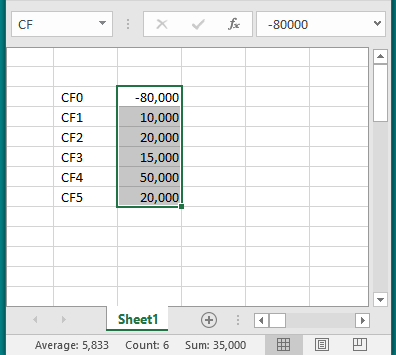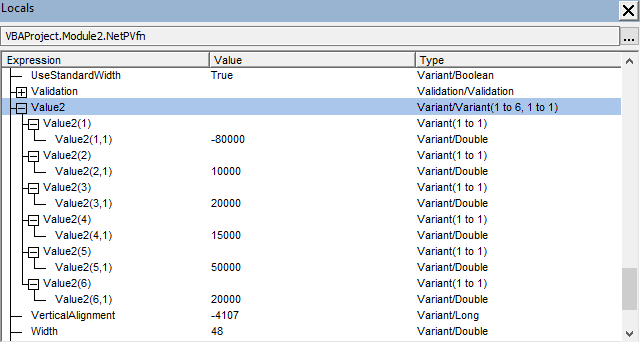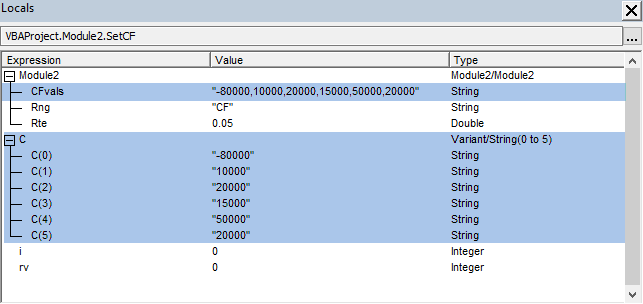# Development of an NetPV engine

In this module we develop a variety of corrections to the Excel NPV function, with three different routines. Each routine includes the cash flow component at time zero:-

• The VBA NPV function, with cash flows from a range object which is assigned to an array
• The WS NPV function with cash flows in a range object
• NPV using first principles with a For...Next loop to read the range object values

The procedures are constructed as possible alternate routines for the analysis engine behind an NPV Estimator UserForm interface described in section 1.2.

## 1. Preliminary

It is well known that the Excel NPV function returns the present value of FUTURE cash flows, and ignores the cash flow component at time zero. In financial modeling, the net present value (NPV) of a project or investment is given by $$\begin{equation} NPV=C_0 + \sum_{t=1}^{n} \frac{C_t}{(1+k)^t} \end{equation}$$ where $C_0$ is the initial cash flow at time zero. The future cash flows at time $t$ are denoted $C_t$ over $n$ periods. $k$ is the periodic discount rate.

### 1.1 The NPV example

Example: ABC Farming Co is considering an investment of $80,000 in a new carrot washing machine, that is expected to generate the following incremental cash inflows:$10,000 (year 1), $20,000 (year 2),$15,000 (year 3), $50,000 (year 4), and$20,000 (year 5). Assume that all cash inflows occur at the end of the year. The discount rate is 5.0% pa. [Source: NPV example.]

Required: Calculate the net present value of the project using equation 1.

Mathematically, the answer to the cash flow example is: $$NPV=-80,000 + \frac{10,000}{(1+0.05)^{1}} + \frac{20,000}{(1+0.05)^{2}}+ \frac{15,000}{(1+0.05)^{3}}+ \frac{50,000}{(1+0.05)^{4}}+ \frac{20,000}{(1+0.05)^{5}} = \17,428$$

The Excel NPV function ignores the $C_0$ component, so equation 1 can be rewritten as $NPV_{xl}$ -

$$\begin{equation} NPV_{\text{xl}}= \sum_{t=1}^{n} \frac{C_t}{(1+k)^t} \end{equation}$$

The NetPV engine: A financial modeling team is developing a demonstration program to return the actual net present value of cash flows with incorporation of the initial cash flow of the project.

The financial model involves both a user interface (section 1.2), and a procedure (analytical engine, described in section 2) to calculate the return value. This module addresses the second part.

### 1.2 The NetPV interface

The UserForm in figure 1 has two Parameters: Discount rate (unlabeled with value 5%), and Cash Flow range (range name CF).Fig 1: NetPV interface - with Parameter and Value frame controls

The sample cash flows worksheet (figure 2) has an m x 1 vector of cash flows (m = 6) with labels one column left. When the cash flow vector is set to the Selection, the name CF appears in the Name Box. Code 6 (described in section 3.1) can be used to establish the CF vector and labels.Fig 2: NetPV sample cash flow - a 6 x 1 vector with name CF

## 2. Calculating NetPV

Three methods are demonstrated. 1. The VBA NPV function; 2. The Excel NPV function, and 3. NPV from first principles.

### 2.0 Module Declaration

Code 1: Module level Declaration
Option Explicit
Option Private Module

' Start edit ==
Const Rte As Double = 0.05
Const Rng As String = "CF"  ' the "Cash Flow range" TextBox / RefEdit control returns a string
Const CFvals As String = "-80000,10000,20000,15000,50000,20000"
' End edit ====


1. Line 2: Option Private Module - all procedures in the module are private and will not appear on the Excel Macro list, or Function list
2. Line 4 to 8: Edit segment - the UserForm is not required in this development phase, so values from the UserForm interface are grouped in the declarations segment to provide ease with future editing
3. Line 7: Const CFvals - represents assignment of the sample cash flow values from figure 1. It is not possible to declare an array constant, so the cash flow vector is written as a string constant, then assigned to an array in a later procedure (code 5 line 84), using the VBA Split function

### 2.1 The VBA NPV function

SYNTAX: NPV function (VBA)


VBA.NPV(Rate As Double, ValueArray() as Double) As Double

The function calculates the net present value (NPV) of a project from a series of future cash flows
(ValueArray) and a discount rate (Rate)



The VBA NPV function requires that the cash flow range object must be assigned to a VBA array of type double. Types such as Variant or even Single will return a Type Mismatch error.

Code 2: Sub procedure NetPVv - NetPV using the vBA NPV function
Sub NetPVv(Disc As Double, CFlow As Range)
Dim CFarray() As Double, CF0 As Double, Tmp As Double
Dim m As Integer, i As Integer

m = CFlow.Rows.Count
ReDim CFarray(1 To m - 1)
CF0 = CFlow(1, 1).Value

For i = 1 To m - 1
CFarray(i) = CFlow(i + 1, 1).Value
Next i

Tmp = VBA.NPV(Disc, CFarray) + CF0
DBPrint (Tmp)

End Sub

Sub RunNetPVv()
Call NetPVv(0.05, Range(Rng))
End Sub


1. Line 10: Procedure declaration - two compulsory arguments including CFlow as type Range. Note: when an object is included in the procedure declaration the Set statement is not required.
2. Line 11: Dynamic array - the cash flow array, named CFarray is a dynamic array as indicated by the empty (). The array is given a dimension in line 15
3. Line 16: CF0 - assign the first cell of Cflow([RowIndex],[ColumnIndex]) CFlow(1, 1).Value returns -80000 to the CF0 variable
4. Line 19: CFarrray(i) - assign the remaining cells of Cflow([RowIndex],[ColumnIndex]) rows 2 to 6 to the CFarray index 1 to 5. The values of CFarray Type Double(1 To 5) are listed in Locals Window in figure 3
5. Line 22: NetPV - call the VBA NPV function and pass arguments Disc, and CFarray (with elements 1 to 5) plus CF0
6. Line 23: DBPrint (described in code 6) - print the NetPV value to the Immediate Window with time stamp (figure 6)
7. Lines 27 to 29: RunNetPV - this procedure is used to pass the arguments to the NetPVv procedure

### 2.2 The Excel NPV function

SYNTAX: NPV function (WS)


NPV(rate,value1,[value2],...)

Application.Npv(Arg1 As Double, Arg2, [Arg3], [Arg4],..., [Arg30]) As Double

The function calculates the net present value (NPV) of a project from a series of future cash flows
(Arg2,...,Arg30) and a discount rate (Arg1)



The WS NPV function cash flows can be individual elements or a Range object

Code 3: Sub procedure NetPVx - NetPV using the Excel NPV function
Sub NetPVx(Disc As Double, CFlow As Range)
Dim CF0 As Double
Dim Tmp As Double
Dim WSF As WorksheetFunction: Set WSF = WorksheetFunction
Dim m As Integer

m = CFlow.Rows.Count

CF0 = CFlow(1, 1).Value
Tmp = WSF.NPV(Disc, CFlow.Range(Cells(2, 1), Cells(m, 1))) + CF0
DBPrint (Tmp)

End Sub

Sub RunNetPVx()
Call NetPVx(0.05, Range(Rng))
End Sub



1. Line 43: WSF - declare WSF as a WorksheetFunction object, then use the Set statement to assign the object to WSF. WSF is used in line 49
The cash flow values are located in the CFlow range object (declared in line 40)
To view the values, expand the CFlow > Value2 expression in the Locals Window (figure 4)Fig 4: Immediate Window - CF0, and CFlow(1)...(6)
2. Value2 is an array of Variants with Type Variant/Variant(1 to 6, 1 to 1)
Each row (dimension 1) of Values2 contains another Variant array for the cells in the Range row
CF0 can also be addressed by CFlow.Value2(1, 1). This is a Variant with sub type Double. Variant/Double in the Type column

### 2.3 NPV from the algebraic formula

In the algebraic form, equation 1 is re-expressed as -

$$\begin{equation} NPV_{\text{loop}}= \sum_{t=0}^{n} \frac{C_t}{(1+k)^t} \end{equation}$$
to sum all of the discounted cash flow, as the main statement in a VBA For...Next loop. See code 4. This is also describes as NPV from first principles.

Code 4: Sub procedure NetPVfn - NetPV with a for...next loop
Sub NetPVfn(Disc As Double, CFlow As Range)
Dim Tmp#, i%

For i = 1 To CFlow.Rows.Count
Tmp = Tmp + CFlow(i, 1) * (1 + Disc) ^ -(i - 1)
Next i
DBPrint (Tmp)

End Sub

Sub RunNetPVfn()
Call NetPVfn(0.05, Range(Rng))
End Sub


1. Line 61: Dim - statement using type declaration characters (TDC's), # for Double, and % for Integer
2. Line 64: NetPV - accumulate the values in Tmp with reference to all of the elements of Cflow([RowIndex],[ColumnIndex]) rows 1 to 6 in CFlow(1)...(6) - see figure 4

## 3. Utility procedures

Two utility programs are described. SetCF is used to set up the cash flow vector in a worksheet, and DBPrint as a short routine to call the Debug.Print statement.

### 3.1 Setting the Cash Flow vector

Code 5: Sub procedure SetCF - set Cash Flow vector
Sub SetCF()
Dim C As Variant
Dim i As Integer, rv As Integer

C = Split(CFvals, ",")

On Error Resume Next
If ActiveSheet.Name(Rng) = Rng Then
rv = MsgBox(Prompt:="The " & Rng & " name already exists" & vbNewLine & _
"Press OK to Overwrite or Relocate at ActiveCell", _
Buttons:=vbOKCancel + vbInformation, _
Title:="Set " & Rng & " sample data series")
If rv = vbCancel Then
Exit Sub
End If
End If
With ActiveCell
.Resize(UBound(C) + 1, 1).Offset(0, 1).Name = Rng
For i = 0 To 5
.Offset(i, 0) = Rng & i
.Offset(i, 1) = C(i)
.Offset(i, 1).NumberFormat = "#,##0"
Next i
End With

End Sub



1. Line 84: use the VBA Split function to the convert the CFvals string, declared at the module level, to elements of the C variant array, figure 5Fig 5: SetCF procedure - Locals Window showing CFvals string variable assigned to the C variant array with base 0

### 3.2 Printing the results

Code 6: Sub procedure DBPrint - Debug.Print routine
Sub DBPrint(Msg As String)
Debug.Print "Time: " & Time & " ===" & vbNewLine & _
"NPV: " & Format(Msg, "Currency") & vbNewLine
End Sub